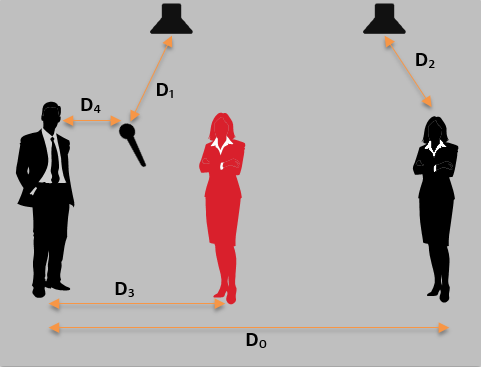# Calculating PAG and NAG

This article explains how to calculate Potential Acoustical Gain (PAG), and Needed Acoustical Gain (NAG). These values are very useful during the design stage of a project, especially in conference rooms that require speech reinforcement.

Needed Acoustical Gain (NAG) helps define a value in decibels the system will require to achieve in order to perform effectively.

Potential Acoustical Gain (PAG) assists in determining the potential maximum amplification in decibels the system can apply before feedback occurs. A Feedback Stability Margin (FSM) of 6dB is often applied to the resulting value.

The target  is to ensure that if we take away PAG from NAG (PAG - NAG), we achieve a value of 0, or even better, positive values. If this calculation results in negative numbers, we know the system will suffer from feedback and people in the room won't be able to hear adequately.

Please note that PAG/NAG calculations are based on the inverse square law but neglect several variables involved in speech reinforcement so are no replacement for more advanced acoustic simulation tools. But if the calculations are applied with care, they can provide a fairly accurate estimation of the expected performance of a sound reinforcement system.

## Calculating PAG and NAG

Definitions:

• D0 - The distance between the talker and the farthest listener.
• D1 - The distance between the source microphone and the nearest loudspeaker.
• D2 - The distance between listener and his/hers nearest loudspeaker.
• D3 - The distance between the talker and the nearest listener.
• D4 - The distance between the talker and the microphone.
• NOM - Number of Open microphones.### PAG formulas

• If NOM equals 1, then PAG  = 20Log((D0 * D1)/(D2 * D4))

• If NOM is greater than 1, then PAG = 20Log((D0 * D1)/(D2 * D4)) - 10LogNOM

• If FSM is applied, then PAG = 20Log((D0 * D1)/(D2 * D4)) - 10LogNOM - 6 dB

### NAG formula

• NAG = 20Log(D0/D3)

## Example

Let's imagine a room with the following dimensions and equipment position:

• D0 = 6 m
• D1 = 4 m
• D2 = 3 m
• D3 = 2 m
• D4 = 1.5 m
• NOM = 3

Applying PAG's formula provides a value of 4 dB:

PAG = 20Log((D0 * D1)/(D2 * D4)) - 10LogNOM - 6 dB

= 20Log((6*4)/(3*1.5)) - 10Log3 - 6 dB = 4 dB

Applying NAG formula provides a value of 9 dB:

NAG = 20Log(D0/D3)

= 20Log(6/2) = 9 dB

If we subtract the results, we end up with a negative value:

PAG-NAG = 4 - 9 = -5 dB

We therefore know that the system won't be able to reach the required amplification in order to work effectively.

But if we reduce the distance between the talker and the microphone (D4) from 1.5 to 0.4, we can review our calculations as follow:

Applying PAG formula provides a value of 15 dB:

PAG = 20Log((D0 * D1)/(D2 * D4)) - 10LogNOM - 6 dB

= 20Log((6*4)/(3*0.4)) - 10Log3 - 6 dB = 15 dB

Applying NAG formula still provides a value of 9 dB:

NAG = 20Log(D0/D3)

= 20Log(6/2) = 9 dB

If we subtract the results, we end up with a positive value:

PAG-NAG = 15 - 9 = 4 dB

We can therefore now predict the system is more likely to perform to the customer's satisfaction.

### Excel calculator

To calculate the formulas mentioned above the following structure should be used in Excel.

PAG (for NOM>1 and FSM=6dB) =20*LOG((Dist0*Dist1)/(Dist2*Dist4))-10*LOG(NOM)-6

NAG =20*LOG(Dist0/Dist3)

Here is a basic spreadsheet calculator for PAG-NAG.

PAG-NAG simple calculator.xlsx